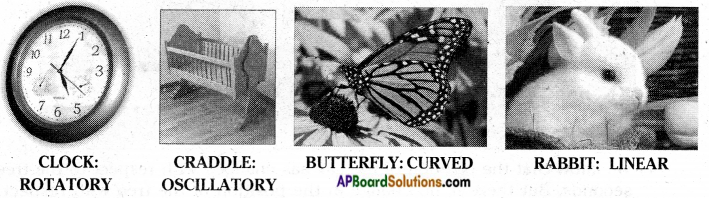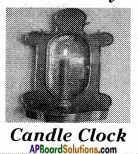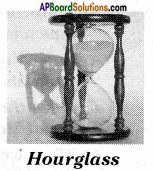AP State Syllabus AP Board 7th Class Science Solutions Chapter 4 Motion and Time Textbook Questions and Answers.

## AP State Syllabus 7th Class Science Solutions 4th Lesson Motion and Time

### 7th Class Science 4th Lesson Motion and Time Textbook Questions and Answers

Question 1.
State whether the following statements are True or False. Rewrite the wrong statements correctly.
a) A body can be at rest and in motion at the same time in relation to the same set of surroundings.
b) A passenger flying in an airplane is at rest with respect to the airport and moving with respect to other passengers.
c) The wheels of a train are in rotatory motion as well as in translatory motion, when it moves.
a)

1. A body can be at rest when it is not moving in relation to the set of surroundings.
2. A body can be at motion when it is in motion at the same time relative to, the set of surroundings.

b)

1. A passenger flying in an airplane is at rest with respect to other passengers.
2. A passenger flying in an airplane is moving with respect to the airport.

c) The wheels of a train are in rotatory motion as well as in translatory motion when it moves.Question 2.
John tied a stone to a string and whirled it around. What type of motion do you find there?
When a stone is tied to a string and whirled it around, we find rotatory motion in it.

Question 3.
What is common to the following? The motion of the propeller of a flying helicopter, the minute hand of a watch, the tape of a cassette recorder.
a) All are examples of translatory motion.
b) All are examples of oscillatory motion.
c) All are examples of rotatory motion.
d) All are examples of periodic motion.
All are examples of rotatory motion.

Question 4.
Which of the following is not an oscillatory motion?
a) Motion of the hammer of an electric bell.
b) Motion of your hands while running.
c) Motion of a child on a see-saw.
d) Motion of a horse pulling a cart.
The motion of a horse pulling a cart is not an oscillatory motion.

Question 5.
Arun completed a 100-meter race in 16s, while Karthik finished it in 13s. Who ran faster?

1. Karthik ran faster.
2. The same distance 100 meter race is completed in 13s by Karthik in a short interval of time, compared to 16s as completed by Arun.
3. So Karthik ran faster.

Question 6.
I. A train runs from New Delhi to Hyderabad. It covers first distance of 420 km in 7 hrs. and next distance of 360 km in 6 hrs.
II. Gopi takes part in a car race. He drives a distance of 70 km each in the first, second and third hours.
Which of the following statements is true?
a) I is an example of uniform motion and II is an example of non-uniform motion.
b) I is an example of non-uniform motion and II is an example of uniform motion.
c) I and II are examples of uniform motion.
d) I and II are examples of non-uniform motion.
a) I is an example of uniform motion and II is an example of non-uniform motion. This statement is not true.
b) I is an example of non uniform motion and II is an example of uniform motion. This statement is true.
c) I and II are examples of uniform motion. This statement is not true.
d) I and II are examples of non-uniform motion. This statement is not true.Question 7.
Write the motion of different parts of a bicycle while it is in motion.
a) the wheel
b) the cycle chain
c) the pedal with its arm
d) the movement of the feet pedaling
e) the movement of the rider along with the bicycle.
a) Rotatory motion
b) Rotatory motion
c) Rotatory motion
d) Oscillatory motion
e) Translatory motion

Question 8.
Which of the following statements is correct?
a) The basic unit of time is second.
b) Every object’s motion is uniform.
c) Two cars move for 5 minutes and 2 minutes respectively. The second car is faster because it takes less time.
d) The speed of a car is expressed in km/h.
a) This statement is correct.
b) This statement is not correct.
c) This statement is not correct.
In the statement distance covered by the cars is not mentioned.
d) This statement is correct.

Question 9.
The basic unit of speed is
A) km/min
B) m/min
C) km/h
D) m/s
D) m/s

Question 10.
The correct relation between speed, distance and time is
A) Speed = distance/time
B) Speed = time/disatnce
C) Speed = distance × time
D) Distance = speed/time
A) Speed = distance/time

Question 11.
The distance between two stations is 240 km. A train takes 4 hrs to cover this distance. Calculate the speed of the train.
Distance between the two stations = 240 km
Time taken by the train to travel this distance = 4 hrs
Speed = $$\frac{\text { Distance travelled }}{\text { Time }}$$ = $$\frac{240}{4}$$ = 60 km/hrQuestion 12.
A train travels at a speed of 180 km/h. How far will it travel in 4 hours?
Speed of the train = 180 km/h
Time of travel = 4 hours
Distance travelled = ?
$$\frac{\text { Distance travelled }}{\text { Time }}$$ = Speed
∴ Distance travelled = speed × time = 180 km/hr × 4h = $$\frac{180 km}{h}$$ × 4h = 720 km

Question 13.
When do you say an object is in rotatory motion?
1) Motion of all particles of a moving object follow a circular path with respect to a fixed centre of axis of rotation.
2) Then the motion of the object is said to be in rotatory motion.

Question 14.
Can an object possess translatory and rotatory motion at the same time? Give an example.

1. An object can possess both translatory and rotatory motions at the same time.
2. The wheel of a cycle during movement will have both rotatory and translatory motions.
3. The wheel rotates (rotatory motion) and at the same time moves forward (Translatory motion).

Question 15.
Make a collection of action pictures showing living and non living things in motion. Paste them neatly in a scrap book. Under each picture write the type of motion the picture shows.Question 16.
In a sewing machine used by tailors, mention the type of motion of sewing machine’s parts when it runs.
a) the wheel b) the needle c) the cloth.
a) The motion of the wheel is rotatory motion.
b) The motion of the needle is oscillatory motion
c) The motion of the cloth is translatory motion.Question 17.
Gather the information about the apparatus used to measure time in olden days.